# JP6605746B2 - Secret coupling system, secret coupling apparatus, secret coupling method, program - Google Patents

Secret coupling system, secret coupling apparatus, secret coupling method, program Download PDF

## Info

Publication number
JP6605746B2
JP6605746B2 JP2018542380A JP2018542380A JP6605746B2 JP 6605746 B2 JP6605746 B2 JP 6605746B2 JP 2018542380 A JP2018542380 A JP 2018542380A JP 2018542380 A JP2018542380 A JP 2018542380A JP 6605746 B2 JP6605746 B2 JP 6605746B2
Authority
JP
Japan
Prior art keywords
column
table
element
σ
secret
Prior art date
Legal status (The legal status is an assumption and is not a legal conclusion. Google has not performed a legal analysis and makes no representation as to the accuracy of the status listed.)
Active
Application number
JP2018542380A
Other languages
Japanese (ja)
Other versions
JPWO2018061800A1 (en
Inventor

Original Assignee

Priority date (The priority date is an assumption and is not a legal conclusion. Google has not performed a legal analysis and makes no representation as to the accuracy of the date listed.)
Filing date
Publication date
Priority to JP2016187782 priority Critical
Priority to JP2016187782 priority
Application filed by 日本電信電話株式会社 filed Critical 日本電信電話株式会社
Priority to PCT/JP2017/033283 priority patent/WO2018061800A1/en
Publication of JPWO2018061800A1 publication Critical patent/JPWO2018061800A1/en
Application granted granted Critical
Publication of JP6605746B2 publication Critical patent/JP6605746B2/en
Active legal-status Critical Current
Anticipated expiration legal-status Critical

• 238000010168 coupling process Methods 0.000 title claims description 123
• 230000001808 coupling Effects 0.000 title claims description 119
• 238000005859 coupling reaction Methods 0.000 title claims description 119
• 238000006467 substitution reaction Methods 0.000 claims description 37
• 238000004364 calculation methods Methods 0.000 claims description 31
• 238000000034 methods Methods 0.000 description 19
• 239000000203 mixtures Substances 0.000 description 9
• 230000000875 corresponding Effects 0.000 description 3
• 238000006243 chemical reaction Methods 0.000 description 1

## Images

•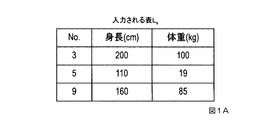•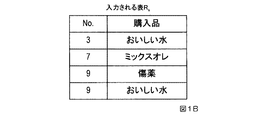••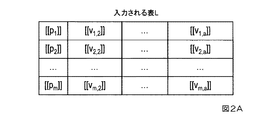•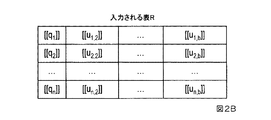•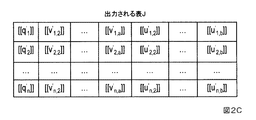•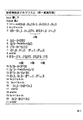•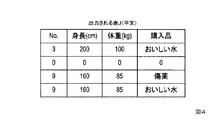•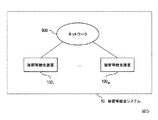•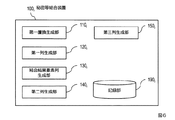•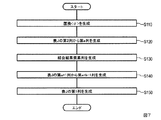•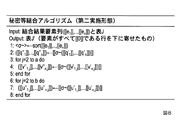•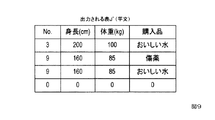•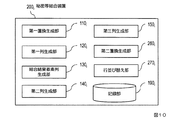•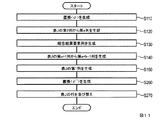•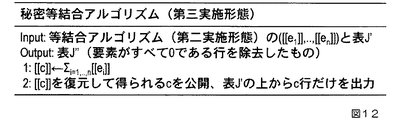•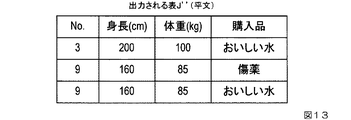•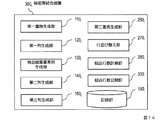•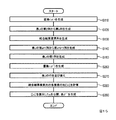## Classifications

• GPHYSICS
• G06COMPUTING; CALCULATING; COUNTING
• G06FELECTRIC DIGITAL DATA PROCESSING
• G06F16/00Information retrieval; Database structures therefor; File system structures therefor
• G06F16/20Information retrieval; Database structures therefor; File system structures therefor of structured data, e.g. relational data
• G06F16/24Querying
• G06F16/245Query processing
• G06F16/2455Query execution
• G06F16/24553Query execution of query operations
• G06F16/24558Binary matching operations
• G06F16/2456Join operations
• GPHYSICS
• G06COMPUTING; CALCULATING; COUNTING
• G06FELECTRIC DIGITAL DATA PROCESSING
• G06F7/00Methods or arrangements for processing data by operating upon the order or content of the data handled
• G06F7/22Arrangements for sorting or merging computer data on continuous record carriers, e.g. tape, drum, disc
• G06F7/24Sorting, i.e. extracting data from one or more carriers, rearranging the data in numerical or other ordered sequence, and rerecording the sorted data on the original carrier or on a different carrier or set of carriers sorting methods in general
• GPHYSICS
• G06COMPUTING; CALCULATING; COUNTING
• G06FELECTRIC DIGITAL DATA PROCESSING
• G06F7/00Methods or arrangements for processing data by operating upon the order or content of the data handled
• G06F7/76Arrangements for rearranging, permuting or selecting data according to predetermined rules, independently of the content of the data
• GPHYSICS
• G09EDUCATION; CRYPTOGRAPHY; DISPLAY; ADVERTISING; SEALS
• G09CCODING OR CIPHERING APPARATUS FOR CRYPTOGRAPHIC OR OTHER PURPOSES INVOLVING THE NEED FOR SECRECY
• G09C1/00Apparatus or methods whereby a given sequence of signs, e.g. an intelligible text, is transformed into an unintelligible sequence of signs by transposing the signs or groups of signs or by replacing them by others according to a predetermined system
• HELECTRICITY
• H04ELECTRIC COMMUNICATION TECHNIQUE
• H04LTRANSMISSION OF DIGITAL INFORMATION, e.g. TELEGRAPHIC COMMUNICATION
• H04L9/00Cryptographic mechanisms or cryptographic arrangements for secret or secure communication
• H04L9/06Cryptographic mechanisms or cryptographic arrangements for secret or secure communication the encryption apparatus using shift registers or memories for block-wise or stream coding, e.g. DES systems or RC4; Hash functions; Pseudorandom sequence generators
• H04L9/0618Block ciphers, i.e. encrypting groups of characters of a plain text message using fixed encryption transformation
• HELECTRICITY
• H04ELECTRIC COMMUNICATION TECHNIQUE
• H04LTRANSMISSION OF DIGITAL INFORMATION, e.g. TELEGRAPHIC COMMUNICATION
• H04L9/00Cryptographic mechanisms or cryptographic arrangements for secret or secure communication
• H04L9/08Key distribution or management, e.g. generation, sharing or updating, of cryptographic keys or passwords
• H04L9/0816Key establishment, i.e. cryptographic processes or cryptographic protocols whereby a shared secret becomes available to two or more parties, for subsequent use
• H04L9/085Secret sharing or secret splitting, e.g. threshold schemes
• HELECTRICITY
• H04ELECTRIC COMMUNICATION TECHNIQUE
• H04LTRANSMISSION OF DIGITAL INFORMATION, e.g. TELEGRAPHIC COMMUNICATION
• H04L9/00Cryptographic mechanisms or cryptographic arrangements for secret or secure communication
• H04L9/08Key distribution or management, e.g. generation, sharing or updating, of cryptographic keys or passwords
• H04L9/0894Escrow, recovery or storing of secret information, e.g. secret key escrow or cryptographic key storage
• HELECTRICITY
• H04ELECTRIC COMMUNICATION TECHNIQUE
• H04LTRANSMISSION OF DIGITAL INFORMATION, e.g. TELEGRAPHIC COMMUNICATION
• H04L2209/00Additional information or applications relating to cryptographic mechanisms or cryptographic arrangements for secret or secure communication H04L9/00
• H04L2209/46Secure multiparty computation, e.g. millionaire problem

## Description

The present invention relates to an equijoining technique for performing equijoining of two tables with a key attribute common to the two tables as a secret while keeping the information contained in the tables secret by secret calculation.

As a method of obtaining a calculation result of a specified calculation without restoring an encrypted numerical value, there is a method called secret calculation (see, for example, Non-Patent Document 1). In the method of Non-Patent Document 1, encryption is performed such that a plurality of pieces of information capable of restoring numerical values are distributed to three secret computing devices, and addition / subtraction, constant sum, multiplication, constant multiplication, The result of logical operation (negation, logical product, logical sum, exclusive logical sum) and data format conversion (integer, binary number) can be kept distributed in three secret computing devices, that is, encrypted. it can. In general, the number of distributions is not limited to 3, and can be W (W is a predetermined constant equal to or greater than 3), and a protocol that realizes secret calculation by cooperative calculation by W secret calculation devices is called a multi-party protocol.

By the way, in many cases of database processing for a table, the data is a plurality of attribute values (values corresponding to the attributes. In the examples of the tables of FIGS. 1A, 1B, and 1C, the attributes No., height, weight, purchase Of records (each row in the table illustrated in FIG. 1A or FIG. 1B) consisting of a set of specific values “3”, “200”, “100”, “delicious water”, etc. Managed in units of tables consisting of sets. One of the important processes in database processing is equijoining. Equal join takes multiple tables as shown in Fig. 1A and Fig. 1B as input, extracts records that have a key attribute value (key attribute value) common to all tables, and puts them sideways It is a calculation that obtains a new table. For example, when the table L s in FIG. 1A and the table R s in FIG. 1B are equi-joined based on a key attribute common to each table (No. in this example), a table J s as shown in FIG. . In relational databases, it is common to divide and manage data into many small tables and perform processing by equally joining tables necessary for use, and equijoining is a very important process.

There is a method of Non-Patent Document 2 as a method of realizing equal join of tables by secret calculation. In the method of Non-Patent Document 2, equijoining of a plurality of tables having overlapping key attribute values is realized.

Koji Senda, Hiroki Hamada, Igarashi Univ., Katsumi Takahashi, "Reconsideration of Lightweight Verifiable 3-Party Secret Function Calculation", In CSS, 2010. Hiroki Hamada, Naoto Toiri, University of Igarashi, "A joint algorithm for secret computation when there are duplicate keys", Symposium on Cryptography and Information Security (SCIS) 2015, IEICE, 2015.

When evaluating computational efficiency assuming that secret computation is realized by a multi-party protocol, the multi-party protocol is a method of performing cooperative computation while communicating among multiple parties (participants), and a general system configuration Then, since the time required for communication is significantly longer than the local calculation performed by each party alone, the local calculation can be regarded as negligible. Therefore, the calculation efficiency is evaluated using a measure of the amount of data communicated (communication amount).

At this time, in the method of Non-Patent Document 2, if the number of rows of two tables to be joined is m and n, and the maximum number of overlapping elements of key attributes to be joined is k, O (k (m + n) log (m + n)) is required, and there is a problem that more communication is required between servers that store data concealed when performing equal coupling. In particular, if there is a possibility that the key attribute “No.” may appear again and again like the attribute “purchased product” in FIG. 1B, the value of k becomes large and the problem becomes obvious. Will be.

Therefore, the present invention provides an equijoining technique for generating one table that is kept secret from two tables that are kept secret while keeping the information contained in the table secret by secret calculation while reducing the traffic. With the goal.

In one embodiment of the present invention, Z N is a finite ring composed of a set of integers from 0 to N (N is an integer of 1 or more), m and n are integers of 1 or more, a and b are integers of 2 or more, p i (1 ≦ i ≦ m), v i, j (1 ≦ i ≦ m, 2 ≦ j ≦ a), q i (1 ≦ i ≦ n), u i, j (1 ≦ i ≦ n, 2 ≦ j ≦ b) is an element of a non-zero finite ring Z N , [[x]] is a secret value of x∈Z N , <π> is a substitution π by secret calculation, and three or more secrets, etc. A secret coupling system that generates a table J of n rows a + b-1 columns from a table L of m rows and a columns and a table R of n rows and b columns, each of which is composed of a coupling device and whose elements are concealed. , First column of table L ([[p 1 ]], .., [[p m ]]), first column of table R ([[q 1 ]], .., [[q n ]) ]) Generated element sequences ([[p 1 ]], .., [[p m ]], [[q 1 ]], .., [[q n ]], [[p 1 ]], .., [[p m ]]) and the first permutation generation means for generating permutation <σ> by stable sorting, and for j = 2, .., a, (1) Element sequence ([],. [],. [, [v1 , j ]], .., [[v m, j ]]) and n []. ., []), the element sequence [[f]] = ([[v 1, j ]], .., [[v m, j ]], [], .., [], [[-v 1, j ]], .., [[-v m, j ]]), and (2) the element sequence [[f ]] To generate an element sequence [[g]] = [[σ ([[f]])]], and (3) calculating the prefix sum of the element sequence [[g]], [g ′]] = PrefixSum ([[g]]), and (4) using the reverse replacement <σ −1 > of the replacement <σ> to the element sequence [[g ′]] [f ']] = [[σ -1 ([[g']])]] is generated, and (5) From the (m + 1) th element to the (m + n) th element in the element sequence [[f ']] Sub-element sequence ([[f ′ m + 1 ]], .., [[f ′ m + n ]]), and the j-th column ([[v ′ 1, j ]],. ., [[v ' n, j ]]) = ([[f' m + 1 ]], .., [[f ' m + n ]]) First column generation means for generating the a-th column from (1), an element sequence ([], .., []) consisting of m [] and n [] [[F1]] = ([], .., [] using an element sequence consisting of ] ([], .., []) , [], .., [], [[-1]], .., [[-1]]) (2) generating an element sequence [[g1]] = [[σ ([[f1]])]] from the element sequence [[f1]] using the replacement <σ>, and (3) By calculating the prefix sum of the element sequence [[g1]], the element sequence [[g1 ']] = PrefixSum ([[g1]]) is generated, and (4) using the above reverse substitution <σ -1 > To generate an element sequence [[f1 ']] = [[σ -1 ([[g1']])]] from the element sequence [[g1 ']], and (5) the element sequence [[f1'] ] From the (m + 1) th element to the (m + n) th element ([[f1'm + 1 ]],., [[F1'm + n ]]) [[e 1 ]], .., [[e n ]]) = ([[f1 ' m + 1 ]], .., [[f1' m + n ]]) Means, j = a + 1, .., a + b−1, the join result element sequence ([[e 1 ]], .., [[e n ]]) and j-a + 1 column ([[u 1, j-a + 1 ]], .., [[u m, j-a + 1 ]]) using the j-th column ([[u ' 1, j-a + 1 ]], .., [[u' n, j-a + 1 ]]) = ([[e 1 ]] × [[u 1, j-a + 1 ]], .., [[e n ]] × [[u n, j−a + 1 ]]) to generate the a + b−1 column from the a + 1 column of the table J Double row generator When the coupling result element rows ([[e 1]], .., [[e n]]) and the first column of the table R ([[q 1]] , .., [[q n]] ) Using the first column ([[q ′ 1 ]], .., [[q ′ n ]]) = ([[e 1 ]] × [[q 1 ]], .., and [[e n ]] × [[q n ]]).

According to the present invention, it is possible to reduce the amount of communication necessary for equal coupling to O ((m + n) log (m + n)), where m and n are the number of rows in two tables that are equally coupled.

It is a figure which shows the example which produces | generates one table | surface by an equijoin from two tables | surfaces (diagram of the table Ls input). The equal coupling from two tables is a diagram illustrating an example of generating one table (FIG tables R s input). It is a figure (diagram of the table J output) which shows the example which produces | generates one table from two tables by an equijoin. It is a figure (diagram of table L inputted) which shows two tables used as an input of a secret coupling algorithm of a first embodiment, and one table used as an output. It is a figure (diagram of table R inputted) which shows two tables used as an input of a secret combination algorithm of a first embodiment, and one table used as an output. FIG. 3 is a diagram (a diagram of an output table J) showing two tables that are inputs and one table that is an output of the secret coupling algorithm of the first embodiment. It is a figure which shows the process sequence of the secret etc. coupling | bonding algorithm of 1st embodiment. It is a figure which shows the example of the table | surface J (plaintext) which is an output result of the secret etc. coupling | bonding algorithm of 1st embodiment. 2 is a block diagram showing a configuration of a secret coupling system 10. FIG. Is a block diagram showing the configuration of a secret such coupling device 100 i. 4 is a flowchart showing an operation of the secret coupling system 10. It is a figure which shows the process sequence of the secret etc. coupling | bonding algorithm of 2nd embodiment. It is a figure which shows the example of the table | surface J '(plaintext) which is an output result of the secret etc. coupling | bonding algorithm of 2nd embodiment. It is a block diagram showing the configuration of a secret such as binding devices 200 i. 3 is a flowchart showing the operation of the secret coupling system 20. It is a figure which shows the process sequence of the secret etc. coupling | bonding algorithm of 3rd embodiment. It is a figure which shows the example of the table | surface J '' (plaintext) which is an output result of the secret etc. coupling algorithm of 3rd embodiment. It is a block diagram showing the configuration of a secret such as binding devices 300 i. 5 is a flowchart showing the operation of the secret coupling system 30.

Hereinafter, embodiments of the present invention will be described in detail. In addition, the same number is attached | subjected to the structure part which has the same function, and duplication description is abbreviate | omitted.

The secret combination algorithm described later is constructed by a combination of existing secret calculation operations. The operations required by these secret etc. combining algorithms are concealment and restoration, addition, multiplication, prefix sum, substitution, reverse substitution, and stable sorting. Before explaining each operation, the necessary definitions and notation are explained.

<Definition and Notation>
Let Z N be a set of integers from 0 to N (N is an integer greater than or equal to 1). That is, Z N = {0,., N}, and Z N forms a finite ring.

Let [[x]] be a value (secret text) where x∈Z N is concealed by encryption or secret sharing. Also, x is referred to as [[x]] plaintext.

[[x]] + [[y]] is added by secret calculation, [[x]], [[y]] are input, and [[x + y]] is output.

[[x]] × [[y]] is multiplication by secret calculation, [[x]], [[y]] are input, and [[x × y]] is output.

PrefixSum ([[x 1 ]], .., [[x n ]]) finds an element sequence called a prefix sum from an element sequence ([[x 1 ]], .., [[x n ]]) This is an operation, and details will be described later.

<σ> represents a replacement σ by secret calculation. Details will be described later.

An element string ([[f 1 ]], .., [[f n ]]) in which each element of the element string f = (f 1 , .., f n ) is concealed is represented as [[f]]. That is, [[f]] = ([[f 1 ]], .., [[f n ]]).

[[σ ([[f] ])]] represents the element sequence obtained by replacing the element sequence [[f]] substitution sigma.

Sort ([[x 1 ]], .., [[x n ]]) takes an element sequence ([[x 1 ]], .., [[x n ]]) as input and sets the replacement <σ> Represents a stable sort to output.

<Operation algorithm>
[Concealment and restoration]
As a method for obtaining [[x]] from x∈Z N (concealment) and a method for obtaining x∈Z N from [[x]] (restoration), specifically, the method of Senda et al. 1) and Shamir's method (reference non-patent document 1).
(Reference Non-Patent Document 1) Shamir, A., “How to share a secret”, Communications of the ACM, Vol.22, No.11, pp.612-613, 1979.

An example of concealment will be described. When the participants in the multi-party protocol are X, Y, and Z, x∈Z N is distributed among a plurality of (for example, three) secret values, and [[x]] is a plurality of secret values x i (i ∈ {1,2,3}). Participants X 1 , Y, Z have a part of the secret value x i (i∈ {1,2,3}) assigned to each person, but the secret value x i (i∈ {1, 2,3}). For example, it is assumed that participant X has set {x 2 , x 3 }, participant Y has set {x 1 , x 3 }, and participant Z has set {x 1 , x 2 }. .

The addition is an algorithm for obtaining [[c]] where c = a + b is concealed when [[a]] and [[b]] of a, b∈Z N are given. Specifically, the method of Ben-Or et al. (Reference non-patent document 2) is known, and communication between participants in the multi-party protocol is unnecessary.

Multiplication is an algorithm for obtaining [[c]] where c = a × b is concealed when [[a]] and [[b]] of a, b∈Z N are given. Specifically, the method of Gennaro et al. (Reference Non-Patent Document 3) can be used. This method requires communication between participants in a multi-party protocol.
(Reference Non-Patent Document 2) Ben-Or, M., Goldwasser, S. and Wigderson, A., “Completeness theorems for non-cryptographic fault-tolerant distributed computation”, Proceedings of the twentieth annual ACM symposium on Theory of computing, ACM, pp. 1-10, 1988.
(Reference 3) Gennaro, R., Rabin, MO and Rabin, T., “ Simplified VSS and fast-track multiparty computations with applications to threshold cryptography”, Proceedings of the seventeenth annual ACM symposium on Principles of distributed computing, ACM, pp.101-111, 1998.

[Prefix-sum]
For a plurality of ordered elements (element sequence), an operation for obtaining the sum of a certain element and all the elements that have appeared so far, and the resulting element sequence are called a prefix sum. That is, element rows ([[x 1]], .., [[x n]]) when a given element sequence with y i obtained by the following formula ([[y 1]], .. , [[y n ]]).

Hereinafter, this operation is represented as ([[y 1 ]], .., [[y n ]]) ← PrefixSum ([[x 1 ]], .., [[x n ]]). By using the method of Ben-Or et al. (Reference Non-Patent Document 2) as the addition algorithm, the prefix sum does not require communication between participants in the multi-party protocol.

[Replace]
The operation of rearranging the ordered elements and its mapping is called replacement. For example, the operation of rearranging (1, 2, 3) to (3, 1, 2) is a replacement with a mapping σ that satisfies σ (1) = 3, σ (2) = 1, and σ (3) = 2. It is regarded. Multiple permutations can be synthesized from the nature of the map. That is, the synthetic substitution σ · π maps the element x to σ (π (x)) with respect to the substitution σ, π.

As one of the methods for realizing the replacement <σ> by the secret calculation, the method of Igarashi et al. (Reference Non-Patent Document 4) can be used.
(Reference Non-Patent Document 4) University of Igarashi, Hiroki Hirota, Ryo Kikuchi, Koji Chida, “Improvement of secret computation radix sort for statistical processing of 1 second of Internet environment response”, Symposium on Cryptography and Information Security (SCIS) 2014, IEICE, 2014.

In this method, for example, when the participants in the multi-party protocol are X, Y, and Z, the replacement σ is a combination of replacements σ XY , σ YZ , and σ ZX that satisfy σ = σ ZX · σ YZ · σ XY . The replacement σ XY is a replacement shared only by the participant X and the participant Y and is not notified to the participant Z. Participant Z obtains a concealment element sequence after replacement by σ XY by redistribution from participant X and participant Y. The same applies to the replacements σ YZ and σ ZX , and any participant does not know the replacement. Therefore, the replacement <σ> by the secret calculation can be executed without all the participants knowing the correspondence.

Here, the replacement <σ> can be regarded as duplicate secret sharing (reference non-patent document 5) that is divided into replacements σ XY , σ YZ , and σ ZX and whose application order is determined. This is a generalization of Shuta et al. (Reference Non-Patent Document 6) in which σ is a random replacement, and the communication cost is linear with respect to the input size.
(Reference Non-Patent Document 5) Ito, M., Saito, A. and Nishizeki, T., “Secret sharing scheme realizing general access structure”, Electronics and Communications in Japan (Part III: Fundamental Electronic Science), Vol.72, No.9, pp.56-64, 1989.
(Reference Non-Patent Document 6) Hiroki Kajita, Dai Igarashi, Koji Senda, Katsumi Takahashi, “Random Replacement Protocol for 3-Party Secret Function Calculation”, Computer Security Symposium (CSS) 2010, Information Processing Society of Japan Computer Security Study Group, 2010.

[Reverse substitution]
Since the permutation is bijective, it has an inverse mapping. Therefore, the inverse mapping of a certain substitution σ is called inverse substitution and is represented by σ −1 . That is, when σ (x) = y, σ −1 (y) = x. In particular, σ −1 · σ is a substitution called the identity substitution that does not change the order.

The method of inverse substitution <σ −1 > by secret calculation is the same as that of substitution. When the substitution <σ> is given as a composition of the substitutions σ XY , σ YZ , σ ZX where σ = σ ZX · σ YZ · σ XY , the reverse substitution is σ -1 = σ XY -1 · σ YZ -1 -Since it becomes σ ZX -1 , it is sufficient to reverse the order of the replacement of each participant X, Y, Z and apply.

[Stable sort]
Sorting that preserves the order of the same elements is called stable sorting. That is, when x i = x j in the stable sort σ that rearranges (x 1 , .., x n ) to (y 1 , .., y n ), σ (x i ) = y u , σ (x j If) = y v , then u <v only when i <j.

An example of the secret calculation method is the method of Igarashi et al. (Reference non-patent document 4). In the following, the output by stable sorting for the element sequence ([[x 1 ]], .., [[x n ]]) is the replacement <σ>, and <σ> ← Sort ([[x 1 ]], .. , [[x n ]]).

<First embodiment>
The secret coupling system that realizes the input and output of the secret coupling algorithm, the processing procedure, the processing cost, and the secret coupling algorithm of the first embodiment will be described below.

In the following, when extracting and handling a table column, it is treated as an element column arranged in the horizontal direction, not as arranged in the vertical direction.

[input]
The two tables to be joined are L and R, respectively (see FIGS. 2A and 2B). The size of the table is m rows and a columns in table L and n rows and b columns in table R (m and n are integers of 1 or more, and a and b are integers of 2 or more). Tables L and R both have key attribute values in the first column, ([[p 1 ]], .., [[p m ]]), ([[q 1 ]], .., [[q n ]]). Tables L and R have attribute values other than key attribute values in the second and subsequent columns, and attribute values of i rows and j columns are [[v i, j ]] (1 ≦ i ≦ m, 2 ≦ j ≦ a) , [[U i, j ]] (1 ≦ i ≦ n, 2 ≦ j ≦ b).

Tables composed of elements obtained by returning the elements of the tables L and R to the plaintext before concealment (hereinafter also referred to as plaintext elements) are denoted as L plain and R plain , respectively. It is assumed that there is no element with the same value in the element column (p 1 , .., p m ) of the key attribute value of table L plain (that is, p 1 , .., p m are different from each other), and table R plain It is assumed that duplicate values may exist in the element sequence (q 1 , .., q n ) of the key attribute value of. Also, the plaintext elements p i (1 ≦ i ≦ m), v i, j (1 ≦ i ≦ m, 2 ≦ j ≦ a), q i (1 ≦ i ≦ n), u of all input elements Let i, j (1 ≦ i ≦ n, 2 ≦ j ≦ b) be a value on the finite ring Z N whose value is not zero. If there is a possibility that the plaintext element contains 0, pre-processing is performed so as not to become 0 by adding and subtracting uniformly, for example. In addition, when there is a possibility that the plaintext element is a value other than the value on the finite ring Z N such as a character string, preprocessing is performed so that the value is on the finite ring Z N.

[output]
The table to be output is J (see FIG. 2C). In Table J, the i-th row (1 ≦ i ≦ n) is ([[q ' i ]], [[v' i, 2 ]], .., [[v ' i, a ]], [[u ' i, 2 ]], .., [[u' i, b ]]) is a table with n rows, and the same value as the key attribute value q i of table R plain is the key attribute value of table L plain Is not in the element sequence (p 1 , .., p m ), the value of the i-th row is all 0, that is, q ′ i = 0 for 2 ≦ α ≦ a and 2 ≦ β ≦ b , v ′ i, α = 0, u ′ i, β = 0. On the other hand, when there is a key attribute value p j of the table L plain with q i = p j for the key attribute value q i of the table R plain , q ′ i = q i , v ′ i, α = v j, α , u ′ i, β = u i, β .

[Processing procedure]
A processing procedure of the secret coupling algorithm of the first embodiment shown in FIG. 3 will be described. At this time, the leftmost numeral in FIG. 3 is used to express Step 1, Step 2, and the like. In order to make the behavior of the algorithm easy to understand, m = 3, a = 3, n = 4, b = 2 and the values of the table L s in FIG. 1A and the table R s in FIG. This will be described by substituting into Table R in FIG. 2B. That is, the description will be made in a plain text state.

First, in step 1, element strings ([[p 1 ]], .., [[p m ]], [[q 1 ]], generated from the key attribute values of the two input tables L and R, .., [[q n ]], [[p 1 ]], .., [[p m ]]) are generated to perform the permutation <σ>.

The replacement <σ> is a replacement that rearranges the following element row in the first row in the order of the second row. 1st to 3rd elements from the left, 4th to 7th, 8th to 10th from the left of the element column in the first row are the key attribute values of the table L s , the key attribute values of the table R s , and the table L s respectively. This is the key attribute value.
(First line) ([[3, [[5, [[9, , , , , 3]], 5]], 9]])
(Second line) ([[3, , 3]], [[5, 5]], , [[9, , , 9]])

Here, for easy understanding of the description, the positional relationship before and after replacement is expressed by using the symbol [[x, [y], z]] to distinguish the same values. However, the processing is actually performed without distinction. [[x and z]] is plaintext corresponding to the attribute values obtained from Table L and [y] is obtained from Table R.

Next, steps 2 to 8 are repeated for each column in Table L (j = 2,..., A). In the example of the table L s in FIG. 1A, there are columns of “height” and “weight” (a = 3). Here, the processing is performed using the column “weight”.

In step 3, the element column ([) in which the j-th column ([[v 1, j ]], .., [[v m, j ]]) and [] in Table L are arranged is arranged. ], .., []) using the element sequence [[f]] = ([[v 1, j ]], .., [[v m, j ]], [], .., [], [[-v 1, j ]], .., [[-v m, j ]]).

The plaintext element string of the element string [[f]] is as follows when the values 100, 19, and 85 of the column “weight” are used. The element string (, , , ) is a plaintext element string of ([], .., []).
([[100, [[19, [[85, , , , , -100]], -19]], -85]])

In Step 4, the element string [[g]] = [[σ ([[f]])]] is generated from the element string [[f]] using the replacement <σ>.

When the plaintext element sequence of the element sequence [[f]] is rearranged by the replacement <σ>, the plaintext element sequence of the element sequence [[g]] is obtained.

([[100, , -100]], [[19, -19]], , [[85, , , -85]])
In step 5, the element string [[g ′]] = PrefixSum ([[g]]) is generated by calculating the prefix sum of the element string [[g]].

The plaintext element sequence of the element sequence [[g ']] obtained by the prefix sum is as follows.
([[100, , 0]], [[19, 0]], , [[85, , , 0]])
By this procedure, it is understood that the plain text [[x is copied to [y] corresponding to the value of the column in Table L.
Also, z]] is a guard in programming, indicating the end point where the value of [[x is copied.

In step 6, the element sequence [[g ′]] = [[σ −1 ([[g ′]])) from the element sequence [[g ′]] using the inverse permutation <σ −1 > of the permutation <σ>. ]] Is generated.

When the plaintext element sequence of the element sequence [[g ′]] is rearranged by reverse substitution <σ −1 >, the plaintext element sequence of the element sequence [[f ′]] is obtained.
([[100, [[19, [[85, , , , , 0]], 0]], 0]])

In step 7, subelement sequences ([[f ′ m + 1 ]],... [[F ′ m + n ] from the ( m + 1 ) -th element to the (m + n) -th element ]]) And take the jth column of table J ([[v'1 , j ]], .., [[v'n , j ]]) = ([[f'm + 1 ]], .. , [[f ' m + n ]]).

The value of the jth column of the generated table J is  of the fourth (= 3 + 1) element to the seventh (= 3 + 4) element of the plaintext element sequence of the element sequence [[f ']]. , , , . Here, the value of the second row of Table J, which is the value of the row that has not been joined, is  in plain text.

The processing from Step 9 to Step 13 is the one in which the processing from Step 3 to Step 7 is applied to a virtual column in which all the values of the columns in Table L are []. Specifically, it is as follows.

In step 9, an element sequence consisting of m [] ([], .., []) and an element sequence consisting of n [] ([ ], .., []) using the element sequence [[f1]] = ([], .., [], [], .., [[0 ]], [[-1]], .., [[-1]]).

The plaintext element sequence of the element sequence [[f1]] is as follows.
([[1, [[1, [[1, , , , , -1]], -1]], -1]])

In Step 10, the element string [[g1]] = [[σ ([[f1]]]]]] is generated from the element string [[f1]] using the replacement <σ>.

When the plaintext element sequence of the element sequence [[f1]] is rearranged by the replacement <σ>, the plaintext element sequence of the element sequence [[g1]] is obtained.
([[1, , -1]], [[1, -1]], , [[1, , , -1]])

In step 11, the element string [[g1 ']] = PrefixSum ([[g1]]) is generated by calculating the prefix sum of the element string [[g1]].

The plaintext element sequence of the element sequence [[g1 ']] obtained by the prefix sum is as follows.
([[1, , 0]], [[1, 0]], , [[1, , , 0]])

In step 12, the element sequence [[f1 ′]] = [[σ −1 ([[g1 ′]]]]] is generated from the element sequence [[g1 ′]] using the inverse permutation <σ −1 >. .

When the plaintext element sequence of the element sequence [[g1 ′]] is rearranged by reverse substitution <σ −1 >, the plaintext element sequence of the element sequence [[f1 ′]] is obtained.
([[1, [[1, [[1, , , , , 0]], 0]], 0]])

In step 13, subelement sequences ([[f1 ′ m + 1 ]],... [[F1 ′ m + n ] from the ( m + 1 ) th element to the (m + n) th element of the element sequence [[f1 ′]]. ]]), And join result element string ([[e 1 ]], .., [[e n ]]) = ([[f1 ' m + 1 ]], .., [[f1' m + n ]]) Is generated. Here, the join result element column ([[e 1 ]], .., [[e n ]]) is an element column indicating whether or not there is a row to be joined to the table L with respect to the row of the table R. , [[E i ]] = [] indicates that there were rows to be joined, and [[e i ]] = [], there were no rows to be joined. Indicates.

The value of the combined result element string to be generated is the ,  of the fourth (= 3 + 1) element to the seventh (= 3 + 4) element of the plaintext element string of the element string [[f1 ']]. 0], , .

The processing from steps 14 to 21 uses the join result element sequence ([[e 1 ]],..., [[E n ]]) to set the values of the rows not joined in the table R to []. It is a process to make.

In steps 15 to 17, the join result element sequence ([[e 1 ]], .., [[e n ]]) and the j-a + 1 column ([[u 1, j-a + 1 ] of Table R) ]], .., [[u m, j-a + 1 ]]) using the j-th column ([[u ' 1, j-a + 1 ]], .., [[u' n, j-a + 1 ]]) = ([[e 1 ]] × [[u 1, j-a + 1 ]], .., [[e n ]] × [[u n, j-a +1 ]]) (j = a + 1,., A + b-1).

In steps 19 to 21, the join result element sequence ([[e 1 ]], .., [[e n ]]) and the first column ([[q 1 ]], .., [[q n ]]) Using the first column of Table J ([[q ' 1 ]], .., [[q' n ]]) = ([[e 1 ]] × [[q 1 ]], .. , [[e n ]] × [[q n ]]).

In the example of the table R s in FIG. 1B, the “mixed” row (second row) that is not combined is 0, and the same value as the original is substituted in the other rows. Therefore, Table J (plain text) as an output result is as shown in FIG.

[Processing cost]
The processing that requires communication in the secret coupling algorithm of the first embodiment is a single stable sort of length 2m + n in step 1 and a replacement of length 2m + n in steps 4 and 10. Similarly, the reverse permutation of length 2m + n in step 6 and step 12 is combined a times, and the multiplication in step 16 and step 20 is bn times.

Communication cost stable sort by Hamada's method (Reference Non-Patent Document 6), O a ((m + n) log ( m + n)), a linear multiplication for the input for substitution and back substitution for that Do not require a constant amount of communication per once. Since the number of columns a and b in each table can be regarded as a constant, the communication amount is O ((m + n) log (m + n)), where m and n are the numbers of rows in the two input tables.

[Secret coupling system]
Hereinafter, the secret coupling system 10 according to the first embodiment will be described with reference to FIGS. FIG. 5 is a block diagram showing the configuration of the secret coupling system 10. The secret coupling system 10 includes W (W is a predetermined integer of 3 or more) secret coupling apparatuses 100 1 ,..., 100 W. The secret coupling devices 100 1 ,..., 100 W are connected to the network 800 and can communicate with each other. The network 800 may be, for example, a communication network such as the Internet or a broadcast communication path. FIG. 6 is a block diagram showing a configuration of the secret coupling device 100 i (1 ≦ i ≦ W). FIG. 7 is a flowchart showing the operation of the secret coupling system 10.

As shown in FIG. 6, the secret coupling device 100 i includes a first replacement generation unit 110 i , a first column generation unit 120 i , a combination result element sequence generation unit 130 i, and a second column generation unit 140 i . , A third row generation unit 150 i and a recording unit 190 i . Each component of the secret coupling device 100 i excluding the recording unit 190 i performs operations required by the secret coupling algorithm, that is, at least concealment, restoration, addition, multiplication, prefix sum, substitution, reverse substitution, and stability. Among the sorts, it is configured so that operations necessary for realizing the function of each component can be executed. In the present invention, specific functional configurations for realizing individual operations are sufficient to execute the algorithms disclosed in Non-Patent Document 1 and Reference Non-Patent Documents 1 to 6, for example. Since this is a conventional configuration, a detailed description thereof will be omitted. The recording unit 190 i is a component that records information necessary for processing of the secret coupling device 100 i .

By the cooperative calculation by the W secret coupling devices 100 i , the secret coupling system 10 implements a secret coupling algorithm that is a multi-party protocol. Therefore, the first replacement generation unit 110 (not shown) of the secret coupling system 10 includes first replacement generation units 110 1 ,..., 110 W , and the first column generation unit 120 (not shown). the first column generating unit 120 1, ..., 120 W is constituted by the coupling result element train generating means 130 (not shown) is coupled result element string generation unit 130 1, ..., it is composed of 130 W, the second column The generation means 140 (not shown) is composed of the second row generation units 140 1 ,..., 140 W , and the third row generation means 150 (not shown) is the third row generation units 150 1 ,. Consists of W.

The secret coupling system 10 receives a table L of m rows and a columns in which each element is concealed and a table R of n rows and b columns as inputs, and performs a secret coupling of the tables L and R to n rows a + Generate Table J in column b-1 (see FIG. 2C). Hereinafter, the operation of the secret coupling system 10 will be described with reference to FIG.

The first permutation generation means 110 includes the first column ([[p 1 ]], .., [[p m ]]) of the table L and the first column ([[q 1 ]],. [[q n ]]) generated element sequence ([[p 1 ]], .., [[p m ]], [[q 1 ]], .., [[q n ]], [[ p 1 ]],.., [[p m ]]) are stably sorted to generate a replacement <σ> (S110). This corresponds to step 1 of the secret coupling algorithm in FIG.

The first column generation means 120 generates the a-th column from the second column of Table J by performing the following processing on j = 2,..., A (S120). This corresponds to steps 2 to 8 of the secret coupling algorithm in FIG.
(1) Element column ([]) with j columns ([[v 1, j ]], .., [[v m, j ]]) and [] arranged in Table L , .., []), the element sequence [[f]] = ([[v 1, j ]], .., [[v m, j ]], [] ,. ., [], [[-v 1, j ]], .., [[-v m, j ]]).
(2) The element sequence [[g]] = [[σ ([[f]])]] is generated from the element sequence [[f]] using the replacement <σ>.
(3) The element string [[g ']] = PrefixSum ([[g]]) is generated by calculating the prefix sum of the element string [[g]].
(4) From element string [[g ']] to element string [[f']] = [[σ -1 ([[g ']])]] using inverse substitution <σ -1 > of substitution <σ> ] Is generated.
(5) Partial element sequence ([[f ' m + 1 ]], .., [[f' m + n ] from the ( m + 1 ) th element to the (m + n) th element of the element sequence [[f ']] ]), And j-th column ([[v'1 , j ]], .., [[v'n , j ]]) = ([[f'm + 1 ]], .., [[f ' m + n ]]).

The combined result element sequence generation means 130 generates a combined result element sequence ([[e 1 ]],... [[E n ]]) by executing the following processing (S130). This corresponds to steps 9 to 13 of the secret coupling algorithm in FIG.
(1) Element sequence consisting of m [] ([], .., []) and element sequence consisting of n [] ([] , .., []) using the element sequence [[f1]] = ([], .., [], [], .., [ ], [[-1]], .., [[-1]]).
(2) The element string [[g1]] = [[σ ([[f1]]]]]] is generated from the element string [[f1]] using the replacement <σ>.
(3) The element string [[g1 ']] = PrefixSum ([[g1]]) is generated by calculating the prefix sum of the element string [[g1]].
(4) The element sequence [[f1 ′]] = [[σ −1 ([[g1 ′]]]]]] is generated from the element sequence [[g1 ′]] using the inverse permutation <σ −1 >.
(5) Partial element sequence ([[f1 ' m + 1 ]], .., [[f1' m + n ] from the ( m + 1 ) th element to the (m + n) th element of the element sequence [[f1 ']] ]), And join result element string ([[e 1 ]], .., [[e n ]]) = ([[f1 ' m + 1 ]], .., [[f1' m + n ] ]).

The second column generation means 140 performs the following processing on j = a + 1, .., a + b-1, thereby performing the processing from the a + 1 column to the a + b-1 column of Table J. Is generated (S140). This corresponds to steps 14 to 18 of the secret coupling algorithm in FIG.
(1) Join result element sequence ([[e 1 ]], .., [[e n ]]) and j-a + 1 column ([[u 1, j-a + 1 ]], .., [[u m, j-a + 1 ]]) using the j-th column ([[u ' 1, j-a + 1 ]], .., [[u' n, j -a + 1 ]]) = ([[e 1 ]] × [[u 1, j-a + 1 ]], .., [[e n ]] × [[u n, j-a + 1 ] ]).

The third column generation means 150 includes the join result element sequence ([[e 1 ]], .., [[e n ]]) and the first column ([[q 1 ]], .., [[ q n ]]) using the first column of table J ([[q ' 1 ]], .., [[q' n ]]) = ([[e 1 ]] × [[q 1 ]], .., [[e n ]] × [[q n ]]) are generated (S150). This corresponds to steps 19 to 21 of the secret coupling algorithm in FIG.

According to the invention of the present embodiment, two of equal coupling key attribute values of the table with a substituent generated as a result of performing a stable sort on main Motoretsu obtained by arranging in a predetermined manner, of one table Even when there is an overlap in key attribute values, it is possible to combine them equally. In the prior art, the maximum number of overlapping elements is k, and one element is replaced with k pieces of non-overlapping information, but this replacement is not necessary, so servers that are required for equi-joining in a state where data is concealed The amount of communication between them can be reduced. More specifically, it is possible to reduce the amount of communication necessary for equal joining to O ((m + n) log (m + n)), where m and n are the number of rows in two tables that are equally joined. Further, it is not necessary to know the maximum number of overlapping elements of key attributes to be combined, which is necessary in the prior art.

<Second embodiment>
In the table J output by the secret coupling algorithm of the first embodiment, the elements of the rows of the table R that are not joined are all [] while maintaining the order of the rows of the input table R. (It is 0 in Table J (plain text) in FIG. 4). For this reason, when the table J is restored, it is understood how many rows of the input table R were not joined. In order to solve this problem, the secret coupling algorithm according to the second embodiment that executes the process of bringing the combined rows onto the table after executing the secret coupling algorithm according to the first embodiment will be described.

The secret coupling system that realizes the input and output of the secret coupling algorithm, the processing procedure, the processing cost, and the secret coupling algorithm of the second embodiment will be described below.

[input]
The join result element sequence ([[e 1 ]],... [[E n ]]) and the table J that is the output result in the secret join algorithm of the first embodiment are input.

[output]
Table J ', in which the rows of Table J are rearranged, is the output. Table J ′ is a table in which the rows in which all elements are [] in the rows of Table J are moved down.

In table J ', the i-th row (1≤i≤n) is ([[q'' i ]], [[v'' i, 2 ]], .., [[v'' i, a ]] , [[u '' i, 2 ]], .., [[u '' i, b ]]). However, [[q '' i ]] is a key attribute value or [], [[v '' i, 2 ]], .., [[v '' i, a ]] are joined from table L Or a-1 [], [[u '' i, 2 ]], .., [[u '' i, b ]] are joined rows from table R or b- This is one [] (see FIG. 8).

[Processing procedure]
FIG. 8 shows a secret coupling algorithm of the second embodiment.

First, in Step 1, a replacement <σ˜> is generated by stably sorting the join result element string ([[e 1 ]],... [[E n ]]).

The replacement <σ ~> is the plaintext element sequence (, ,  of the join result element sequence ([[e 1 ]], .., [[e n ]]) of the first embodiment. , ) is a replacement that rearranges the following element row in the order of the second row.
(Line 1) (, , , )
(Second line) (, , , )

In step 2, the first column ([[q ' n ]]) from the first column ([[q' 1 ]], .., [[q ' n ]]) of the table J is replaced with the substitution <σ ~>. '' 1 ]], .., [[q '' n ]]) = [[σ ~ ([[q ' 1 ]], .., [[q' n ]])]].

In step 4, using the substitution <σ ~>, the j-th column of table J ′ from the j-th column ([[v ′ 1, j ]], .., [[v ′ n, j ]]) of table J ([[v '' 1, j ]], .., [[v '' n, j ]]) = [[σ ~ ([[v ' 1, j ]], .., [[v' n , j ]])]]] (j = 2, .., a).

In step 7, using the substitution <σ ~>, the jth column of table J ([[u'1 , j-a + 1 ]], .., [[u'n , j-a + 1 ]]) Column j of table J '([[u'' 1, j-a + 1 ]], .., [[u'' n, j-a + 1 ]]) = [[σ ~ ([[ u ' 1, j-a + 1 ]], .., [[u' n, j-a + 1 ]])]] (j = a + 1, .., a + b-1) .

In step 2, step 4, and step 7, the rows whose elements are all [] are moved down, and the table J 'is output. Therefore, the table J ′ (plain text) as an output result is as shown in FIG.

If an ascending sort is used as the stable sort used in step 1, the top and bottom of the output line are inverted, and the line with all the elements [] can be moved up.

[Processing cost]
The communication cost by the secret coupling algorithm of the second embodiment is O (nlog (n)) necessary for stable sorting.

[Secret coupling system]
The secret coupling system 20 according to the second embodiment will be described below with reference to FIGS. Secret such coupling system 20, W pieces secret such binding apparatus 100 1 of (W 3 or more predetermined integer), ..., instead of including a 100 W, W number of secret such binding apparatus 200 1, ..., 200 W Is different from the secret coupling system 10 in that FIG. 10 is a block diagram showing a configuration of the secret coupling device 200 i (1 ≦ i ≦ W). FIG. 11 is a flowchart showing the operation of the secret coupling system 20.

As shown in FIG. 10, the secret coupling device 200 i is different from the secret coupling device 100 i in that it further includes a second substitution generation unit 260 i and a row rearrangement unit 270 i . The second replacement generation unit 260 i and the row rearrangement unit 270 i are also configured to be able to execute an operation necessary for realizing the function among operations required for the secret combination algorithm. .

The second replacement generation means 260 (not shown) of the secret coupling system 20 includes second replacement generation units 260 1 ,..., 260 W , and the line rearrangement means 270 (not shown) 270 1 ,... 270 W.

The secret coupling system 20 receives an m-row a-column table L in which each element is concealed and an n-row b-column table R as inputs, and n-row a + b- A table J ′ having n rows and a + b−1 columns is generated from the table J having one column. Hereinafter, the operation of the secret coupling system 20 will be described with reference to FIG. Since the processing from S110 to S150 is the same as that of the secret coupling system 10, S260 and S270 will be described.

The second permutation generation means 260 generates permutation <σ˜> by stably sorting the join result element string ([[e 1 ]],... [[E n ]]) (S260). This corresponds to step 1 of the secret coupling algorithm in FIG.

The row rearranging means 270 uses the replacement <σ ~> to change the first column of the table J ′ from the first column ([[q ′ 1 ]],... [[Q ′ n ]]) of the table J. ([[q '' 1 ]], .., [[q '' n ]]) = [[σ ~ ([[q ' 1 ]], .., [[q' n ]])]] Generate jth column of table J ′ from j column ([[v ′ 1, j ]], .., [[v ′ n, j ]]) for j = 2, .., a j column ([[v '' 1, j ]], .., [[v '' n, j ]]) = [[σ ~ ([[v ' 1, j ]], .., [[v ' n, j ]])]]], and for j = a + 1, .., a + b-1, the jth column of table J ([[u' 1, j-a + 1 ]] , .., [[u ' n, j-a + 1 ]]) to column j ([[u'' 1, j-a + 1 ]], .., [[u'' n, j-a + 1 ]]) = [[σ ~ ([[u ' 1, j-a + 1 ]], .., [[u' n, j-a + 1 ]])]] Generate (S270). This corresponds to steps 2 to 8 of the secret coupling algorithm in FIG.

According to the invention of the present embodiment, since the newly required communication cost is O (nlog (n)), the entire communication cost is O ((m + n) log (m + n)). can do.

<Third embodiment>
The table J ′ output by the secret coupling algorithm of the second embodiment includes a row that has not been combined as a row in which all elements are []. However, when the number of joined rows may be known to the participant, only the rows that are joined and moved up from the table J ′ may be output. After executing the secret coupling algorithm of the second embodiment, a secret coupling algorithm of the third embodiment that outputs a table including only the joined rows will be described.

The secret coupling system that realizes the input algorithm of the third embodiment, such as the secret coupling algorithm, the output, the processing procedure, the processing cost, and the secret coupling algorithm will be described below.

[input]
A join result element sequence ([[e 1 ]],... [[E n ]]) in the secret coupling algorithm of the first embodiment and a table J ′ that is an output result of the secret coupling algorithm of the second embodiment Is the input.

[output]
A table obtained by deleting a row from table J 'from which all elements are [] in the row of table J' is output as table J ''.

[Processing procedure]
FIG. 12 shows a secret coupling algorithm according to the third embodiment.

First, in step 1, the sum [[c]] of each element of the join result element string ([[e 1 ]],... [[E n ]]) is calculated in order to count the joined rows.

In step 2, c obtained by restoring [[c]] obtained in step 1 is disclosed, and a table J ″ is generated by extracting c rows from the table J ′.

In step 2, a table J ″ from which rows whose elements are all [] is deleted is output. Therefore, the table J ″ (plain text) as the output result is as shown in FIG.

[Processing cost]
The communication cost by the secret coupling algorithm of the third embodiment is only a constant amount O (1) necessary for one disclosure.

[Secret coupling system]
Hereinafter, the secret coupling system 30 according to the third embodiment will be described with reference to FIGS. 14 to 15. The secret coupling system 30 includes W secret coupling devices 300 1 ,..., 300 W instead of including W (W is a predetermined integer of 3 or more) secret coupling devices 200 1 ,. Is different from the secret coupling system 20 in that FIG. 14 is a block diagram showing a configuration of the secret coupling device 300 i (1 ≦ i ≦ W). FIG. 15 is a flowchart showing the operation of the secret coupling system 30.

As shown in FIG. 14, the secret coupling device 300 i is different from the secret coupling device 200 i in that it further includes a combined row number calculation unit 380 i and a combined row number disclosure unit 390 i . The combined row number calculation unit 380 i and the combined row number disclosure unit 390 i are also configured so as to be able to execute operations necessary for realizing the function among operations necessary for the secret combination algorithm. Yes.

The combined row number calculating means 380 (not shown) of the secret combination system 30 is composed of combined row number calculating sections 380 1 ,..., 380 W , and the combined row number disclosing means 390 (not shown) is a combined row. few public section 390 1, ..., composed of 390 W.

The secret coupling system 30 receives an m-row a-column table L in which each element is concealed and an n-row b-column table R as inputs, and n-row a + b- Generate table j 'with n rows and a + b-1 columns from table J with 1 column, and delete rows from table J' with all elements [], so that c rows a + b- Generate a single-column table J ''. Hereinafter, the operation of the secret coupling system 30 will be described with reference to FIG. Since the processing from S110 to S270 is the same as that of the secret coupling system 20, S380 and S390 will be described.

The combined row number calculation means 380 calculates the sum [[c]] of each element of the combined result element string ([[e 1 ]],... [[E n ]]) (S380). This corresponds to step 1 of the secret coupling algorithm in FIG.

The combined row number disclosure unit 390 discloses c obtained by restoring [[c]], and generates a table J ″ obtained by extracting c rows from the table J ′ (S390). This corresponds to step 2 of the secret coupling algorithm in FIG.

According to the invention of the present embodiment, since the newly required communication cost is O (1), the entire communication cost can be executed with O ((m + n) log (m + n)). it can.

<Supplementary note>
The apparatus of the present invention includes, for example, a single hardware entity as an input unit to which a keyboard or the like can be connected, an output unit to which a liquid crystal display or the like can be connected, and a communication device (for example, a communication cable) capable of communicating outside the hardware entity Can be connected to a communication unit, a CPU (Central Processing Unit, may include a cache memory or a register), a RAM or ROM that is a memory, an external storage device that is a hard disk, and an input unit, an output unit, or a communication unit thereof , A CPU, a RAM, a ROM, and a bus connected so that data can be exchanged between the external storage devices. If necessary, the hardware entity may be provided with a device (drive) that can read and write a recording medium such as a CD-ROM. A physical entity having such hardware resources includes a general-purpose computer.

The external storage device of the hardware entity stores a program necessary for realizing the above functions and data necessary for processing the program (not limited to the external storage device, for example, reading a program) It may be stored in a ROM that is a dedicated storage device). Data obtained by the processing of these programs is appropriately stored in a RAM or an external storage device.

In the hardware entity, each program stored in an external storage device (or ROM or the like) and data necessary for processing each program are read into a memory as necessary, and are interpreted and executed by a CPU as appropriate. . As a result, the CPU realizes a predetermined function (respective component requirements expressed as the above-described unit, unit, etc.).

The present invention is not limited to the above-described embodiment, and can be appropriately changed without departing from the spirit of the present invention. In addition, the processing described in the above embodiment may be executed not only in time series according to the order of description but also in parallel or individually as required by the processing capability of the apparatus that executes the processing. .

As described above, when the processing functions in the hardware entity (the apparatus of the present invention) described in the above embodiments are realized by a computer, the processing contents of the functions that the hardware entity should have are described by a program. Then, by executing this program on a computer, the processing functions in the hardware entity are realized on the computer.

The program describing the processing contents can be recorded on a computer-readable recording medium. As the computer-readable recording medium, for example, any recording medium such as a magnetic recording device, an optical disk, a magneto-optical recording medium, and a semiconductor memory may be used. Specifically, for example, as a magnetic recording device, a hard disk device, a flexible disk, a magnetic tape or the like, and as an optical disk, a DVD (Digital Versatile Disc), a DVD-RAM (Random Access Memory), a CD-ROM (Compact Disc Read Only). Memory), CD-R (Recordable) / RW (ReWritable), etc., magneto-optical recording medium, MO (Magneto-Optical disc), etc., semiconductor memory, EEP-ROM (Electronically Erasable and Programmable-Read Only Memory), etc. Can be used.

The program is distributed by selling, transferring, or lending a portable recording medium such as a DVD or CD-ROM in which the program is recorded. Furthermore, the program may be distributed by storing the program in a storage device of the server computer and transferring the program from the server computer to another computer via a network.

A computer that executes such a program first stores, for example, a program recorded on a portable recording medium or a program transferred from a server computer in its own storage device. When executing the process, this computer reads the program stored in its own storage device and executes the process according to the read program. As another execution form of the program, the computer may directly read the program from a portable recording medium and execute processing according to the program, and the program is transferred from the server computer to the computer. Each time, the processing according to the received program may be executed sequentially. Also, the program is not transferred from the server computer to the computer, and the above-described processing is executed by a so-called ASP (Application Service Provider) type service that realizes the processing function only by the execution instruction and result acquisition. It is good. Note that the program in this embodiment includes information that is used for processing by an electronic computer and that conforms to the program (data that is not a direct command to the computer but has a property that defines the processing of the computer).

In this embodiment, a hardware entity is configured by executing a predetermined program on a computer. However, at least a part of these processing contents may be realized by hardware.

The foregoing descriptions of the embodiments of the present invention have been presented for purposes of illustration and description. There is no intention to be exhaustive and there is no intention to limit the invention to the exact form disclosed. Variations and variations are possible from the above teachings. The embodiments are provided in order to provide the best illustration of the principles of the present invention, and to enable those skilled in the art to adapt the present invention in various embodiments and in a variety of ways suitable for practical use. It was chosen and expressed in order to make it possible to use it with modification. All such variations and variations are within the scope of the present invention as defined by the appended claims, which are construed in accordance with the breadth that is impartially impartially and fairly.

## Claims (8)

1. Z N is a finite ring consisting of a set of integers from 0 to N (N is an integer of 1 or more), m and n are integers of 1 or more, a and b are integers of 2 or more, p i (1 ≦ i ≦ m , Where p 1 , .., p m are different from each other), v i, j (1 ≦ i ≦ m, 2 ≦ j ≦ a), q i (1 ≦ i ≦ n), u i, j (1 ≦ i ≦ n, 2 ≦ j ≦ b) is a non-zero element of finite ring Z N ,
Let [[x]] denote the secret value of x∈Z N , <π> denote the substitution π by secret calculation,
A secret that generates a table J of n rows a + b-1 columns from a table L of m rows and a columns and a table R of n rows and b columns, which is composed of three or more secret coupling devices, and each element is concealed An equal coupling system,
The first column of the table L ([[p 1 ]], .., [[p m ]]), the first column of the table R ([[q 1 ]], .., [[q n ]] ) Generated element sequences ([[p 1 ]], .., [[p m ]], [[q 1 ]], .., [[q n ]], [[p 1 ]],. ., [[p m ]]) and first replacement generating means for generating a replacement <σ> by stable sorting;
j = 2, .., a
(1) The element column ([] in which the j-th column ([[v 1, j ]], .., [[v m, j ]]) and [] in Table L are arranged ], .., []) using the element sequence [[f]] = ([[v 1, j ]], .., [[v m, j ]], [], .., [], [[-v 1, j ]], .., [[-v m, j ]]))
(2) Generate an element sequence [[g]] = [[σ ([[f]])]] from the element sequence [[f]] using the replacement <σ>,
(3) By calculating the prefix sum of the element sequence [[g]], an element sequence [[g ']] = PrefixSum ([[g]]) is generated,
(4) From the element sequence [[g ′]] to the element sequence [[f ′]] = [[σ −1 ([[g ′]]] using the inverse permutation <σ −1 > of the permutation <σ> )]]
(5) Partial element sequence ([[f ' m + 1 ]], .., [[f' m + n ] from the ( m + 1 ) th element to the (m + n) th element of the element sequence [[f ']] ]]), And j-th column ([[v'1 , j ]], .., [[v'n , j ]]) = ([[f'm + 1 ]] ,. ., [[f ' m + n ]]) to generate a column a from column 2 of Table J;
(1) Element sequence consisting of m [] ([], .., []) and element sequence consisting of n [] ([] , .., []) using the element sequence [[f1]] = ([], .., [], [], .., [ ], [[-1]], .., [[-1]])
(2) Generate an element sequence [[g1]] = [[σ ([[f1]]]]] from the element sequence [[f1]] using the replacement <σ>,
(3) By calculating the prefix sum of the element sequence [[g1]], the element sequence [[g1 ']] = PrefixSum ([[g1]]) is generated,
(4) Generate element sequence [[f1 ']] = [[σ -1 ([[g1']])]] from the element sequence [[g1 ']] using the reverse permutation <σ -1 > And
(5) Partial element sequence ([[f1 ′ m + 1 ]], .., [[f1 ′ m + n ) from the (m + 1) th element to the (m + n) th element of the element sequence [[f1 ′]] ]]), And join result element string ([[e 1 ]], .., [[e n ]]) = ([[f1 ' m + 1 ]], .., [[f1' m + n ]]) To generate a combined result element sequence generation means;
For j = a + 1, .., a + b−1, the join result element sequence ([[e 1 ]], .., [[e n ]]) and the j-a in Table R +1 rows ([[u 1, j- a + 1]], .., [[u m, j-a + 1]]) j-th column of the table J using ([[u '1, j-a + 1 ]], .., [[u ' n, j-a + 1 ]]) = ([[e 1 ]] × [[u 1, j-a + 1 ]], .., [[e n ]] × [[u n, j−a + 1 ]]) to generate the second column generation from the a + 1th column of the table J to the a + b−1 column Means,
The combination result element string ([[e 1 ]], .., [[e n ]]) and the first column of the table R ([[q 1 ]], .., [[q n ]]) Using the first column ([[q ' 1 ]], .., [[q' n ]]) = ([[e 1 ]] × [[q 1 ]], .., [[ e n ]] × [[q n ]]))
Including secret etc. combined system.
2. Z N is a finite ring consisting of a set of integers from 0 to N (N is an integer of 1 or more), m and n are integers of 1 or more, a and b are integers of 2 or more, p i (1 ≦ i ≦ m , Where p 1 , .., p m are different from each other), v i, j (1 ≦ i ≦ m, 2 ≦ j ≦ a), q i (1 ≦ i ≦ n), u i, j (1 ≦ i ≦ n, 2 ≦ j ≦ b) is a non-zero element of finite ring Z N ,
Let [[x]] denote the secret value of x∈Z N , <π> denote the substitution π by secret calculation,
It is composed of three or more secret coupling devices, and each element is concealed from a table L of m rows a columns and a table R of n rows b columns using the secret coupling system according to claim 1 and n rows A secret coupling system that generates a table J ′ with a + b−1 columns,
Second replacement generation means for generating a replacement <σ ~> by stably sorting the combined result element string ([[e 1 ]],... [[E n ]]);
Using the substitution <σ ~>, the first column ([[q ′ 1 ]) of the table J ′ from the first column ([[q ′ 1 ]],... [[Q ′ n ]]) of the table J is used. '' 1 ]], .., [[q '' n ]]) = [[σ ~ ([[q ' 1 ]], .., [[q' n ]])]] Column j of the table J from the j-th column ([[v ′ 1, j ]], .., [[v ′ n, j ]]]) for = 2, .., a ([[v '' 1, j ]], .., [[v '' n, j ]]) = [[σ ~ ([[v ' 1, j ]], .., [[v' n , j ]])]]], and j = a + 1, .., a + b−1, the j-th column of table J ([[u ′ 1, j-a + 1 ]], .., [[u ' n, j-a + 1 ]]) to j-th column ([[u'' 1, j-a + 1 ]], .., [[u'' n, j-a + 1 ]]) = [[σ ~ ([[u ' 1, j-a + 1 ]], .., [[u' n, j-a + 1 ]])]] A line rearrangement means to be generated;
Including secret etc. combined system.
3. Z N is a finite ring consisting of a set of integers from 0 to N (N is an integer of 1 or more), m and n are integers of 1 or more, a and b are integers of 2 or more, p i (1 ≦ i ≦ m , Where p 1 , .., p m are different from each other), v i, j (1 ≦ i ≦ m, 2 ≦ j ≦ a), q i (1 ≦ i ≦ n), u i, j (1 ≦ i ≦ n, 2 ≦ j ≦ b) is a non-zero element of finite ring Z N ,
Let [[x]] denote the secret value of x∈Z N , <π> denote the substitution π by secret calculation,
It is composed of 3 or more secret coupling devices, and each element is concealed from a table L of m rows and a columns and a table R of n rows and b columns using the secret coupling system according to claim 2. A secret coupling system that generates a table J '' with a + b-1 columns,
A joined row number calculating means for calculating a sum [[c]] of each element of the joined result element string ([[e 1 ]],... [[E n ]]);
Publishing the c obtained by restoring the sum [[c]], and joining row number publishing means for generating the table J '' obtained by extracting c rows from the table J ';
Including secret etc. combined system.
4. Z N is a finite ring consisting of a set of integers from 0 to N (N is an integer of 1 or more), m and n are integers of 1 or more, a and b are integers of 2 or more, p i (1 ≦ i ≦ m , Where p 1 , .., p m are different from each other), v i, j (1 ≦ i ≦ m, 2 ≦ j ≦ a), q i (1 ≦ i ≦ n), u i, j (1 ≦ i ≦ n, 2 ≦ j ≦ b) is a non-zero element of finite ring Z N ,
Let [[x]] denote the secret value of x∈Z N , <π> denote the substitution π by secret calculation,
Secret consisting of 3 or more secret coupling devices that generate n rows a + b-1 columns table J from m rows a columns table L and n rows b columns table R where each element is concealed A secret coupling device in an equal coupling system,
The first column of the table L ([[p 1 ]], .., [[p m ]]), the first column of the table R ([[q 1 ]], .., [[q n ]] ) Generated element sequences ([[p 1 ]], .., [[p m ]], [[q 1 ]], .., [[q n ]], [[p 1 ]],. ., [[p m ]])) to generate a replacement <σ> by stable sorting,
j = 2, .., a
(1) The element column ([] in which the j-th column ([[v 1, j ]], .., [[v m, j ]]) and [] in Table L are arranged ], .., []) using the element sequence [[f]] = ([[v 1, j ]], .., [[v m, j ]], [], .., [], [[-v 1, j ]], .., [[-v m, j ]]))
(2) Generate an element sequence [[g]] = [[σ ([[f]])]] from the element sequence [[f]] using the replacement <σ>,
(3) By calculating the prefix sum of the element sequence [[g]], an element sequence [[g ']] = PrefixSum ([[g]]) is generated,
(4) From the element sequence [[g ′]] to the element sequence [[f ′]] = [[σ −1 ([[g ′]]] using the inverse permutation <σ −1 > of the permutation <σ> )]]
(5) Partial element sequence ([[f ' m + 1 ]], .., [[f' m + n ] from the ( m + 1 ) th element to the (m + n) th element of the element sequence [[f ']] ]]), And j-th column ([[v'1 , j ]], .., [[v'n , j ]]) = ([[f'm + 1 ]] ,. ., [[f ' m + n ]]) to generate a first column from the second column of Table J,
(1) Element sequence consisting of m [] ([], .., []) and element sequence consisting of n [] ([] , .., []) using the element sequence [[f1]] = ([], .., [], [], .., [ ], [[-1]], .., [[-1]])
(2) Generate an element sequence [[g1]] = [[σ ([[f1]]]]] from the element sequence [[f1]] using the replacement <σ>,
(3) By calculating the prefix sum of the element sequence [[g1]], the element sequence [[g1 ']] = PrefixSum ([[g1]]) is generated,
(4) Generate element sequence [[f1 ']] = [[σ -1 ([[g1']])]] from the element sequence [[g1 ']] using the reverse permutation <σ -1 > And
(5) Partial element sequence ([[f1 ′ m + 1 ]], .., [[f1 ′ m + n ) from the (m + 1) th element to the (m + n) th element of the element sequence [[f1 ′]] ]]), And join result element string ([[e 1 ]], .., [[e n ]]) = ([[f1 ' m + 1 ]], .., [[f1' m + n ]]) To generate a combined result element string generation unit;
For j = a + 1, .., a + b−1, the join result element sequence ([[e 1 ]], .., [[e n ]]) and the j-a in Table R +1 rows ([[u 1, j- a + 1]], .., [[u m, j-a + 1]]) j-th column of the table J using ([[u '1, j-a + 1 ]], .., [[u ' n, j-a + 1 ]]) = ([[e 1 ]] × [[u 1, j-a + 1 ]], .., [[e n ]] × [[u n, j-a + 1 ]]) to generate a second a + b−1 column from the a + 1 th column of Table J A column generator;
The combination result element string ([[e 1 ]], .., [[e n ]]) and the first column of the table R ([[q 1 ]], .., [[q n ]]) Using the first column ([[q ' 1 ]], .., [[q' n ]]) = ([[e 1 ]] × [[q 1 ]], .., [[ e n ]] × [[q n ]]) for generating a third column,
Including secret coupling devices.
5. Z N is a finite ring consisting of a set of integers from 0 to N (N is an integer of 1 or more), m and n are integers of 1 or more, a and b are integers of 2 or more, p i (1 ≦ i ≦ m , Where p 1 , .., p m are different from each other), v i, j (1 ≦ i ≦ m, 2 ≦ j ≦ a), q i (1 ≦ i ≦ n), u i, j (1 ≦ i ≦ n, 2 ≦ j ≦ b) is a non-zero element of finite ring Z N ,
Let [[x]] denote the secret value of x∈Z N , <π> denote the substitution π by secret calculation,
A secret coupling system including three or more secret coupling devices, including a first replacement generation unit, a first column generation unit, a combination result element column generation unit, a second column generation unit, and a third column generation unit. A secret-like join method for generating an n-row a + b-1 column table J from an m-row a-column table L and an n-row b-column table R, wherein each element is concealed,
The first permutation generation means includes a first column ([[p 1 ]], .., [[p m ]]) of the table L and a first column ([[q 1 ]],. ., [[q n ]]) generated element sequences ([[p 1 ]], .., [[p m ]], [[q 1 ]], .., [[q n ]], [[p 1 ]], .., [[p m ]]) are first sorted to generate a replacement <σ> by stable sorting;
For the first row generating means, j = 2, .., a,
(1) The element column ([] in which the j-th column ([[v 1, j ]], .., [[v m, j ]]) and [] in Table L are arranged ], .., []) using the element sequence [[f]] = ([[v 1, j ]], .., [[v m, j ]], [], .., [], [[-v 1, j ]], .., [[-v m, j ]]))
(2) Generate an element sequence [[g]] = [[σ ([[f]])]] from the element sequence [[f]] using the replacement <σ>,
(3) By calculating the prefix sum of the element sequence [[g]], an element sequence [[g ']] = PrefixSum ([[g]]) is generated,
(4) From the element sequence [[g ′]] to the element sequence [[f ′]] = [[σ −1 ([[g ′]]] using the inverse permutation <σ −1 > of the permutation <σ> )]]
(5) Partial element sequence ([[f ' m + 1 ]], .., [[f' m + n ] from the ( m + 1 ) th element to the (m + n) th element of the element sequence [[f ']] ]]), And j-th column ([[v'1 , j ]], .., [[v'n , j ]]) = ([[f'm + 1 ]] ,. ., [[f ' m + n ]]) to generate a first column from the second column of Table J;
The combined result element sequence generating means
(1) Element sequence consisting of m [] ([], .., []) and element sequence consisting of n [] ([] , .., []) using the element sequence [[f1]] = ([], .., [], [], .., [ ], [[-1]], .., [[-1]])
(2) Generate an element sequence [[g1]] = [[σ ([[f1]]]]] from the element sequence [[f1]] using the replacement <σ>,
(3) By calculating the prefix sum of the element sequence [[g1]], the element sequence [[g1 ']] = PrefixSum ([[g1]]) is generated,
(4) Generate element sequence [[f1 ']] = [[σ -1 ([[g1']])]] from the element sequence [[g1 ']] using the reverse permutation <σ -1 > And
(5) Partial element sequence ([[f1 ′ m + 1 ]], .., [[f1 ′ m + n ) from the (m + 1) th element to the (m + n) th element of the element sequence [[f1 ′]] ]]), And join result element string ([[e 1 ]], .., [[e n ]]) = ([[f1 ' m + 1 ]], .., [[f1' m + n ]]) To generate a join result element sequence generation step;
The second column generation means, for j = a + 1, .., a + b−1, the combined result element sequence ([[e 1 ]], .., [[e n ]]) and Using the j-a + 1 column ([[u 1, j-a + 1 ]],... [[U m, j-a + 1 ]]) of the table R, the j-th column of the table J Sequence ([[u ' 1, j-a + 1 ]], .., [[u' n, j-a + 1 ]]) = ([[e 1 ]] × [[u 1, j-a +1 ]], .., [[e n ]] × [[u n, j-a + 1 ]])), the a + b−1 A second column generation step for generating a column;
The third column generating means includes the combination result element sequence ([[e 1 ]],.., [[E n ]]) and the first column ([[q 1 ]],. [[q n ]]) using the first column ([[q ' 1 ]], .., [[q' n ]]) = ([[e 1 ]] × [[q 1 ]], .., [[e n ]] × [[q n ]]))
Perform a secret etc. join method.
6. Z N is a finite ring consisting of a set of integers from 0 to N (N is an integer of 1 or more), m and n are integers of 1 or more, a and b are integers of 2 or more, p i (1 ≦ i ≦ m , Where p 1 , .., p m are different from each other), v i, j (1 ≦ i ≦ m, 2 ≦ j ≦ a), q i (1 ≦ i ≦ n), u i, j (1 ≦ i ≦ n, 2 ≦ j ≦ b) is a non-zero element of finite ring Z N ,
Let [[x]] denote the secret value of x∈Z N , <π> denote the substitution π by secret calculation,
Consists of three or more secret coupling devices, first replacement generation means, first column generation means, combination result element sequence generation means, second column generation means, third column generation means, second replacement generation means and row By using a secret coupling system including a sorting means, the table L of the m-row a column, the table R of the n-row b column, and the table J 'of the n-row a + b-1 column where each element is concealed A secret coupling method to generate,
After generating the table J from the table L and the table R by the secret coupling method according to claim 5,
A second replacement generation step in which the second replacement generation means generates a replacement <σ ~> by stably sorting the combination result element string ([[e 1 ]],... [[E n ]]). When,
The row rearranging means uses the replacement <σ ~> to change the table J ′ from the first column ([[q ′ 1 ]],... [[Q ′ n ]]) of the table J. 1st column ([[q '' 1 ]], .., [[q '' n ]]) = [[σ ~ ([[q ' 1 ]], .., [[q' n ]]) ]], And for j = 2, .., a, the j-th column of table J ([[v'1 , j ]], .., [[v'n , j ]]) Column j of table J '([[v'' 1, j ]], .., [[v'' n, j ]]) = [[σ ~ ([[v' 1, j ]] ,. , [[v ' n, j ]])]], and j = a + 1, .., a + b−1, the j-th column ([[u ′ 1, j -a + 1 ]], .., [[u ' n, j-a + 1 ]]) to the j-th column ([[u'' 1, j-a + 1 ]],. ., [[u '' n, j-a + 1 ]]) = [[σ ~ ([[u ' 1, j-a + 1 ]], .., [[u' n, j-a + 1 ]])]] line sorting step to generate,
Perform a secret etc. join method.
7. Z N is a finite ring consisting of a set of integers from 0 to N (N is an integer of 1 or more), m and n are integers of 1 or more, a and b are integers of 2 or more, p i (1 ≦ i ≦ m , Where p 1 , .., p m are different from each other), v i, j (1 ≦ i ≦ m, 2 ≦ j ≦ a), q i (1 ≦ i ≦ n), u i, j (1 ≦ i ≦ n, 2 ≦ j ≦ b) is a non-zero element of finite ring Z N ,
Let [[x]] denote the secret value of x∈Z N , <π> denote the substitution π by secret calculation,
Consists of three or more secret coupling devices, first replacement generation means, first column generation means, combination result element sequence generation means, second column generation means, third column generation means, second replacement generation means and row Using a secret coupling system including a sorting means, a joined row number calculating means, and a joined row number disclosing means, each element is concealed in an m-row a-column table L, an n-row b-column table R to c A secret join method that generates a table J '' with row a + b-1 columns,
After generating the table J ′ from the table L and the table R by the secret coupling method according to claim 6,
A combined row number calculating step in which the combined row number calculating means calculates a sum [[c]] of each element of the combined result element string ([[e 1 ]],... [[E n ]]); ,
The joined row number publishing means publishes the c obtained by restoring the sum [[c]], and publishes the joined row number publication that generates the table J ″ obtained by extracting c rows from the top of the table J ′. Steps,
Perform a secret etc. join method.
8.   The program for functioning a computer as a secret coupling | bonding apparatus which comprises the secret coupling | bonding system of any one of Claim 1 thru | or 3.
JP2018542380A 2016-09-27 2017-09-14 Secret coupling system, secret coupling apparatus, secret coupling method, program Active JP6605746B2 (en)

## Priority Applications (3)

Application Number Priority Date Filing Date Title
JP2016187782 2016-09-27
JP2016187782 2016-09-27
PCT/JP2017/033283 WO2018061800A1 (en) 2016-09-27 2017-09-14 Secret equi-join system, secret equi-join device, secret equi-join method, and program

## Publications (2)

Publication Number Publication Date
JPWO2018061800A1 JPWO2018061800A1 (en) 2019-06-24
JP6605746B2 true JP6605746B2 (en) 2019-11-13

# Family

## Family Applications (1)

Application Number Title Priority Date Filing Date
JP2018542380A Active JP6605746B2 (en) 2016-09-27 2017-09-14 Secret coupling system, secret coupling apparatus, secret coupling method, program

## Country Status (6)

US (1) US20190228010A1 (en)
EP (1) EP3522137A4 (en)
JP (1) JP6605746B2 (en)
CN (1) CN109791741A (en)
AU (1) AU2017338060B2 (en)
WO (1) WO2018061800A1 (en)

## Families Citing this family (2)

* Cited by examiner, † Cited by third party
Publication number Priority date Publication date Assignee Title
WO2019244756A1 (en) * 2018-06-20 2019-12-26 日本電信電話株式会社 Secret combination system, method, secret calculation device, and program
WO2020036127A1 (en) * 2018-08-13 2020-02-20 日本電信電話株式会社 Secret join information generation system, secret join system, methods therefor, secret calculation device, and program

## Family Cites Families (2)

* Cited by examiner, † Cited by third party
Publication number Priority date Publication date Assignee Title
JP5907902B2 (en) * 2013-01-21 2016-04-26 日本電信電話株式会社 Table equijoin system by secret calculation, method
JP6321216B2 (en) * 2015-01-15 2018-05-09 日本電信電話株式会社 Matrix / key generation device, matrix / key generation system, matrix combination device, matrix / key generation method, program

## Also Published As

Publication number Publication date
US20190228010A1 (en) 2019-07-25
CN109791741A (en) 2019-05-21
AU2017338060B2 (en) 2020-06-25
EP3522137A4 (en) 2020-05-20
WO2018061800A1 (en) 2018-04-05
JPWO2018061800A1 (en) 2019-06-24
EP3522137A1 (en) 2019-08-07
AU2017338060A1 (en) 2019-04-11

## Similar Documents

Publication Publication Date Title
Bost et al. Machine learning classification over encrypted data.
US20160358165A1 (en) Cryptographically concealing amounts transacted on a ledger while preserving a network&#39;s ability to verify the transaction
Ananth et al. Optimizing obfuscation: avoiding Barrington's theorem
Dunning et al. Privacy preserving data sharing with anonymous ID assignment
Mayberry et al. Efficient Private File Retrieval by Combining ORAM and PIR.
Cheon et al. Homomorphic computation of edit distance
Amy et al. Estimating the cost of generic quantum pre-image attacks on SHA-2 and SHA-3
Jha et al. Towards practical privacy for genomic computation
Gutub et al. Counting-based secret sharing technique for multimedia applications
Goodrich et al. Privacy-preserving access of outsourced data via oblivious RAM simulation
Zhang et al. An image encryption scheme based on the MLNCML system using DNA sequences
Zhong Privacy-preserving algorithms for distributed mining of frequent itemsets
AU2010256869B2 (en) Generating obfuscated data
Gentry et al. Private database access with he-over-oram architecture
Doerner et al. Scaling ORAM for secure computation
Giannotti et al. Privacy-preserving mining of association rules from outsourced transaction databases
US8074068B2 (en) Secret sharing device, method, and program
Bracken et al. A few more quadratic APN functions
Goodrich et al. Privacy-preserving group data access via stateless oblivious RAM simulation
Yang et al. Privacy-preserving computation of Bayesian networks on vertically partitioned data
JP5411994B2 (en) Secret sharing system, secret sharing apparatus, secret sharing method, secret sorting method, secret sharing program
Rao Extractors for low-weight affine sources
Chen et al. A fast image encryption scheme with a novel pixel swapping-based confusion approach
CN104426973B (en) A kind of cloud database encryption method, system and device
Wang et al. Improved division property based cube attacks exploiting algebraic properties of superpoly

## Legal Events

Date Code Title Description
A521 Written amendment

Free format text: JAPANESE INTERMEDIATE CODE: A523

Effective date: 20190305

A621 Written request for application examination

Free format text: JAPANESE INTERMEDIATE CODE: A621

Effective date: 20190305

TRDD Decision of grant or rejection written
A01 Written decision to grant a patent or to grant a registration (utility model)

Free format text: JAPANESE INTERMEDIATE CODE: A01

Effective date: 20191015

A61 First payment of annual fees (during grant procedure)

Free format text: JAPANESE INTERMEDIATE CODE: A61

Effective date: 20191016

R150 Certificate of patent or registration of utility model

Ref document number: 6605746

Country of ref document: JP

Free format text: JAPANESE INTERMEDIATE CODE: R150